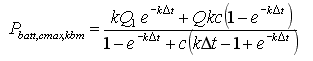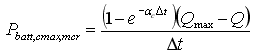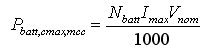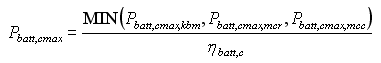# HOMER Grid 1.2

 Navigation: HOMER's Calculations How HOMER Calculates the Maximum Battery Charge PowerIn each time step, HOMER calculates the maximum amount of power that the storage bank can absorb. It uses this maximum charge power when making decisions such as whether the storage bank can absorb all available surplus renewable power or how much surplus power a cycle charging generator should produce. The maximum charge power varies from one time step to the next according to its state of charge and its recent charge and discharge history.

HOMER imposes three separate limitations on the storage bank's maximum charge power. The first limitation comes from the kinetic storage model. As described in the Kinetic Battery Model section of the help, the maximum amount of power that can be absorbed by the two-tank system is given by the following equation:where: Q1 = the available energy [kWh] in the storage at the beginning of the time step Q = the total amount of energy [kWh] in the storage at the beginning of the time step c = the storage capacity ratio [unitless] k = the storage rate constant [h-1] Δt = the length of the time step [h]

The second limitation relates to the maximum charge rate of the Storage Component, which is the A/Ah value on the Storage page. The storage charge power corresponding to this maximum charge rate is given by the following equation:where: αc is the storage's maximum charge rate [A/Ah] Qmax is the total capacity of the storage bank [kWh]

The third limitation relates to the Storage Component's maximum charge current, which also appears on the Storage page. The maximum storage bank charge power corresponding to this maximum charge current is given by the following equation:where: Nbatt = the number of batteries in the storage bank Imax = the storage's maximum charge current [A] Vnom = the storage's nominal voltage [V]

HOMER sets the maximum storage charge power equal to the least of these three values, assuming each applies after charging losses, hence:where ηbatt,c is the storage charge efficiency.